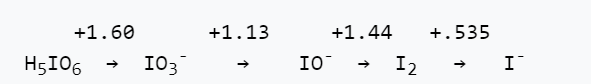# 4.8: Problems

1. Balance the following redox reactions, adding H2O and H+ (or OH-) as needed. Predict for each one whether the reaction would become more or less spontaneous at higher pH.

(a) $$\ce{MnO4^{-}_{(aq)} + N2O_{(g)} = Mn^{2+}_{(aq)} + NO3^{-}_{(aq)}}$$ (in acid)

(b) $$\ce{Cr2O7^{2-}_{(aq)} + S2O3^{2-}_{(aq)} = Cr2O3_{(s)} + SO4^{2-}_{(aq)}}$$ (in base)

(c) $$\ce{H2O2_{(aq)} + HI_{(aq)} = I3^{-}_{(aq)}}$$ (in acid)

(d) $$\ce{HOBr_{(aq)} = HBr_{(aq)} + HBrO2_{(aq)}}$$ (in acid)

(e) $$\ce{C12H22O11 (sucrose, aq) + ClO3^{-}_{(aq)} = HCO3^{-}_{(aq)} + Cl^{-}_{(aq)}}$$ (in base)

2. Silver metal is not easily oxidized, and does not react with oxygen-saturated water. However, when excess NaCN is added to a suspension of silver particles, some silver dissolves. If oxygen is removed (e.g., by bubbling nitrogen through the solution), the dissolution reaction stops. Write a balanced equation for the dissolution reaction (hint: it is a redox reaction).

3. The standard potentials for the Fe3+/Fe2+ and Cl-/Cl2 couples are +0.77 and +1.36 V. Calculate the cell potential of a redox flow battery that has Fe3+/Fe2+ and Cl-/Cl2 solutions on the two sides (both containing 1.0 M HCl as the electrolyte). The pressure of Cl2 gas on the chlorine side is 0.2 atm, and the concentrations of Fe2+ and Fe3+ on the iron side are both 0.10 M.

4. The Latimer potential diagram for iodine (in acidic solutions) is given below.(a) What is the potential for the IO3-/I- redox couple?

(b) Construct a Frost diagram and identify any species that are unstable with respect to disproportionation.

5. The Latimer diagram for nitrogen in acidic solutions is shown below:(a) Write a balanced half reaction for reduction of NO to NH4+ in acid.

(b) What is the value of Eo for the half reaction in part (a)?

(c) Is this half reaction more thermodynamically favorable in acid or in base? Explain.

(d) All the nitrogen-containing molecules and ions listed above are kinetically stable, but only three are thermodynamically stable with respect to disproportionation in acid. Which ones are they?

6. Referring to the Pourbaix diagram for Mn below:

(a) Write out the balanced half reaction corresponding to the line separating Mn2+ and Mn2O3. Use your answer to calculate the slope of the line (give units).

(b) Is Mn metal stable in water at any pH? If so, in what range of pH?

(c) What spontaneous reaction would you expect for an aqueous solution of MnO4- at pH 6?

(d) Describe an electrochemical procedure (specifying pH and potential) for making Mn3O4(s) from aqueous Mn2+.

(e) Label the regions of the diagram that correspond to corrosion and passivation of Mn metal.7. The Pourbaix diagram for copper is shown below.(a) Write a balanced half-reaction that corresponds to the boundary between the Cu2O(s) and Cu(OH)2(s) regions of the diagram.

(b) What is the slope of the line (do not try to measure it from the graph!) that connects the Cu2+(aq) and Cu2O(s) regions? Explain your reasoning.

(c) Over what pH range (if any) is copper metal stable in contact with pure water?

(d) Thermodynamically, the reaction between coppper and oxygen is spontaneous at pH 7. Would you expect copper to corrode or to be passivated against corrosion in aerated water at neutral pH? Explain your reasoning.

(e) Some recent studies have suggested the use of Cu2O as a water splitting photocatalyst. Would you expect Cu2O to be stable in the presence of the oxygen formed in the reaction? Explain your reasoning.